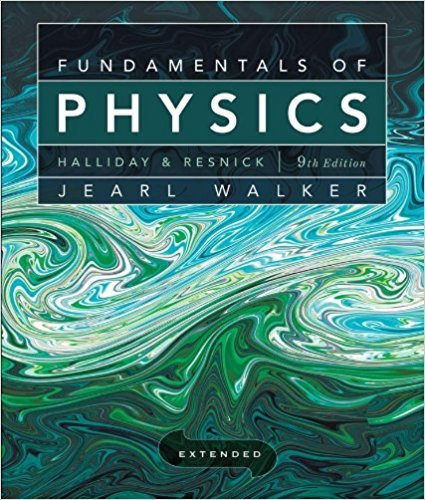×
×

# Solved: In Fig. lS-44a, two identical rectangular rods ofISBN: 9780470469088 189

## Solution for problem 59 Chapter 18

Fundamentals of Physics Extended | 9th Edition

• Textbook Solutions
• 2901 Step-by-step solutions solved by professors and subject experts
• Get 24/7 help from StudySoup virtual teaching assistantsFundamentals of Physics Extended | 9th Edition

4 5 1 392 Reviews
23
3
Problem 59

In Fig. lS-44a, two identical rectangular rods of metal are welded end to end, with a temperature of TI = OC on the left side and a temperature of T2 = lOOC on the right side. In 2.0 min, 10 J is conducted at a constant rate from the right side to the left side. How much time would be required to conduct 10 J if the rods were welded side to side as in Fig. 18-44b?

Step-by-Step Solution:
Step 1 of 3

1/11/16 • Phy206–EverythingbutMechanicsandE&M • SpringSemester2016...

Step 2 of 3

Step 3 of 3

##### ISBN: 9780470469088

The full step-by-step solution to problem: 59 from chapter: 18 was answered by , our top Physics solution expert on 12/27/17, 08:15PM. Since the solution to 59 from 18 chapter was answered, more than 225 students have viewed the full step-by-step answer. The answer to “In Fig. lS-44a, two identical rectangular rods of metal are welded end to end, with a temperature of TI = OC on the left side and a temperature of T2 = lOOC on the right side. In 2.0 min, 10 J is conducted at a constant rate from the right side to the left side. How much time would be required to conduct 10 J if the rods were welded side to side as in Fig. 18-44b?” is broken down into a number of easy to follow steps, and 77 words. Fundamentals of Physics Extended was written by and is associated to the ISBN: 9780470469088. This textbook survival guide was created for the textbook: Fundamentals of Physics Extended, edition: 9. This full solution covers the following key subjects: . This expansive textbook survival guide covers 37 chapters, and 3413 solutions.

Unlock Textbook Solution